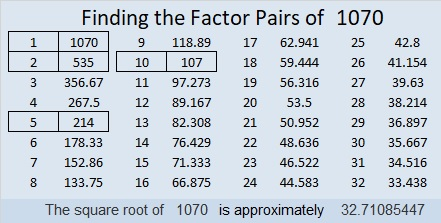# 1070 A Complicated Logic Mystery

This mystery level puzzle requires complicated logic just to get started. If you get stuck, ask yourself these questions:
What two clues must use both 5’s?
What two clues must, therefore, use both 6’s?
What do you know about clue 36 because it shares a common factor with 24?
What two clues must use both 3’s?
What do you now know about the common factor of clues 8 and 24?
What do you also now know about the common factor of clues 36 and 24?Print the puzzles or type the solution in this excel file: 12 factors 1063-1072

Here is a little information about the number 1070:

• 1070 is a composite number.
• Prime factorization: 1070 = 2 × 5 × 107
• The exponents in the prime factorization are 1, 1, and 1. Adding one to each and multiplying we get (1 + 1)(1 + 1)(1 + 1) = 2 × 2 × 2 = 8. Therefore 1070 has exactly 8 factors.
• Factors of 1070: 1, 2, 5, 10, 107, 214, 535, 1070
• Factor pairs: 1070 = 1 × 1070, 2 × 535, 5 × 214, or 10 × 107
• 1070 has no square factors that allow its square root to be simplified. √1070 ≈ 32.710851070 is the hypotenuse of a Pythagorean triple:
642-856-1070 which is (3-4-5) times 214